Courses

# NCERT Solutions(Part- 2)- Comparing Quantities Class 8 Notes | EduRev

## Class 8 : NCERT Solutions(Part- 2)- Comparing Quantities Class 8 Notes | EduRev

The document NCERT Solutions(Part- 2)- Comparing Quantities Class 8 Notes | EduRev is a part of the Class 8 Course Class 8 Mathematics by VP Classes.
All you need of Class 8 at this link: Class 8

Question 1. A shop gives 20% discount. What would the sale price of each of these be?

(a) A dress marked at Rs 120  (b) A pair of shoes marked at Rs 750  (c) A bag marked at Rs 250

Solution:

(a) Marked price of the dress = Rs 120

Discount rate = 20%

∴ Discount = 20% of Rs 120

= Rs 20/100 * 120

= Rs 2 * 12 = Rs 24

∴ Sale price of the dress = [Marked price] – [Discount]

= Rs 120 – Rs 24

= Rs 96

(b) Marked price of the pair of shoes = Rs 750

Discount rate = 20%

∴ Discount = 20% of Rs 750

= Rs 20/100 * 750

= Rs 2 * 75 = Rs 150

Now,

Sale price of the pair of shoes = [Marked price] – [Discount]

= Rs 750 – Rs 150 = Rs 600

(c) Marked price of the bag = Rs 250

Discount rate = 20%

∴ Discount = 20% of Rs 250

= Rs 20/100 * 250

= Rs 2 * 25 = Rs 50

∴ Sale price of the bag = [Marked price] – [Discount]

= Rs 250 – Rs 50 = Rs 200

Question 2. A table marked at Rs 15,000 is available for Rs 14,400. Find the discount given and the discount per cent.

Solution:

Marked price of the table = Rs 15000

Sale price of the table = Rs 14400

∴ Discount = [Marked price] – [Sale price]

= [Rs 15000] – [Rs 14400]

= Rs 600

Discount per cent = Discount/Marked price x  100

= 600/15000 * 100%

= (2 * 2)%

= 4%

Question 3. An almirah is sold at Rs 5,225 after allowing a discount of 5%. Find its marked price.

Solution:

Sale price of the almirah = Rs 5225

Discount rate = 5%

Since, Discount = 5% of marked price

∴ [Marked price] – Discount = Sale price

or [Marked price] – 5/100 * [Marked price] = Sale price

or Marked price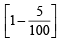= Sale price

or Marked price 95/100 = Sale price (= Rs 5225)

or Marked price = Rs 5225 * 100/95

= Rs 5500

Question 1. Find selling price (SP) if a profit of 5% is made on

(a) a cycle of Rs 700 with Rs 50 as overhead charge.

(b) a lawn mower bought at Rs 1150 with Rs 50 as transportation charges.

(c) a fan bought for Rs 560 and expenses of Rs 40 made on its repairs.

Solution:

(a) ∵ Total cost price = Rs 700 + Rs 50 (Overhead expenses)

= Rs 750

Profit = 5% of Rs 750

= Rs 5/100 * Rs 750 = Rs 5 * 15/2

= Rs 75/2 = Rs 37.50

Now, SP = CP + Profit

= Rs 750 + Rs 37.50

= Rs 787.50

(b) ∵ Total cost price = Rs 1150 + Rs 50 (Overhead expenses)

= Rs 1200

∴ Profit = 5% of Rs 1200

= 5/100 * Rs 1200

= Rs 5 * 12 = Rs 60

Now, SP = CP + Profit

= Rs 1200 + Rs 60

= Rs 1260

(c) ∵ Total cost price = Rs 560 + Rs 40 (Overhead expenses)

= Rs 600

∴ Profit = 5% of Rs 600

= 5/100 * Rs 600

= Rs 5 * 6 = Rs 30

Now, SP = CP + Profit

= Rs 600 + Rs 30

= Rs 630

Question 2. A shopkeeper bought two TV sets at Rs 10,000 each. He sold one at a profit 10% and the other at a loss of 10%. Find whether he made an overall profit or loss.

Solution: Total CP of both TV’s = 2 x Rs 10,000

= Rs 20,000

For 1st TV: CP = Rs 10,000.

Profit rate = 10%

∴ Profit = 10% or Rs 10,000

= 10/100 * Rs 10,000 = Rs 1000

∴ SP = Rs 10,000 + Rs 1000 = Rs 11,000

For 2nd TV: CP = Rs 10,000

Loss rate = 10%

∴ Loss = 10% of Rs 10,000

= 10/100 * Rs 10,000 = Rs 1000

∴ SP = CP – Loss

= Rs 10,000 – Rs 1000 = Rs 9000

Now, Total SP of both the TV’s = Rs 11,000 + Rs 9000

= Rs 20,000

∵ Cost price = Selling price

∴ There is no overall profit or loss.

Question 1. Find the buying price of each of the following when 5% ST is added on the purchase of

(a) A towel at Rs 50

(b) Two bars of soap at Rs 35 each

(c) 5 kg of flour at Rs 15 per kg

Solution:

(a) Cost of the towel = Rs 50

∴ Sales tax on the towel = 5% of Rs 50

= Rs 5/100 * 50 = Rs 2.5

Thus, the buying price of the towel = Rs 50 + Rs 2.5

= Rs 52.5

(b) ∵ Cost of the two soap bars = (Rs 35) * 2 = Rs 70

∴ Sales tax = 5% of Rs 70

= Rs 5/100 * 70 = Rs 3.5

Thus, the buying price = Rs 70 + Rs 3.5

= Rs 73.5

(c) ∵ Cost of 5 kg of flour = Rs 15 * 5 = Rs 75

∴ Sales tax = 5% of Rs 75

= Rs 5/100 * 75

= Rs 375/100 = Rs 3.75

Thus, the buying price = Rs 75 + Rs 3.75

= Rs 78.75

Question 2. If 8% VAT is included in the prices, find the original price of

(a) A TV bought for Rs 13,500  (b) A shampoo bottle bought for Rs 180

Solution:

(a) Cost of TV including VAT = Rs 13500

Rate of VAT = 8%

Let the original price = Rs x

∴  x + 8% of x = Rs 13,500

or  x + 8/100 x =  Rs 13500

or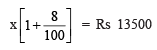or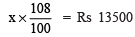or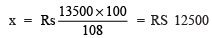Thus, the original price = Rs 12500.

(b) Let original price = Rs x

∴ Price including VAT = Rs x + 8% of x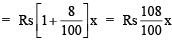But the original price + VAT = Rs 180

∴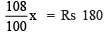or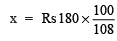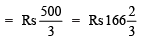Thus, the original price = Rs 166 2/3

Question 1. Two times a number is a 100% increase in the number. If we take half the number, what would be the decrease in per cent?

Solution: Let the number be x.

∴ Decrease in the number = 1/2 of x

= 1/2 of x = x/2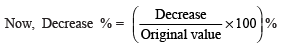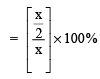= 1/2 *100 % = 50%

Question 2. By what per cent is Rs 2,000 less than Rs 2,400? Is it the same as the per cent by which Rs 2,400 is more than Rs 2,000?

Solution:

Case I

Here 2000 < 2400.

i.e. 2400 is reduced to 2000.

∴ Decrease = 2400 – 2000 = 400

Thus Decrease % =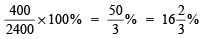Thus, Decrease % =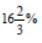Case II

2000 is increased to 2400.

∴ Increase = 2400 – 2000 = 400

∴ Decrease % = 400/2000 x 100% = 20%

Thus, Decrease % = 20%

Obviously, they are not same.

Offer running on EduRev: Apply code STAYHOME200 to get INR 200 off on our premium plan EduRev Infinity!

93 docs|16 tests

,

,

,

,

,

,

,

,

,

,

,

,

,

,

,

,

,

,

,

,

,

;